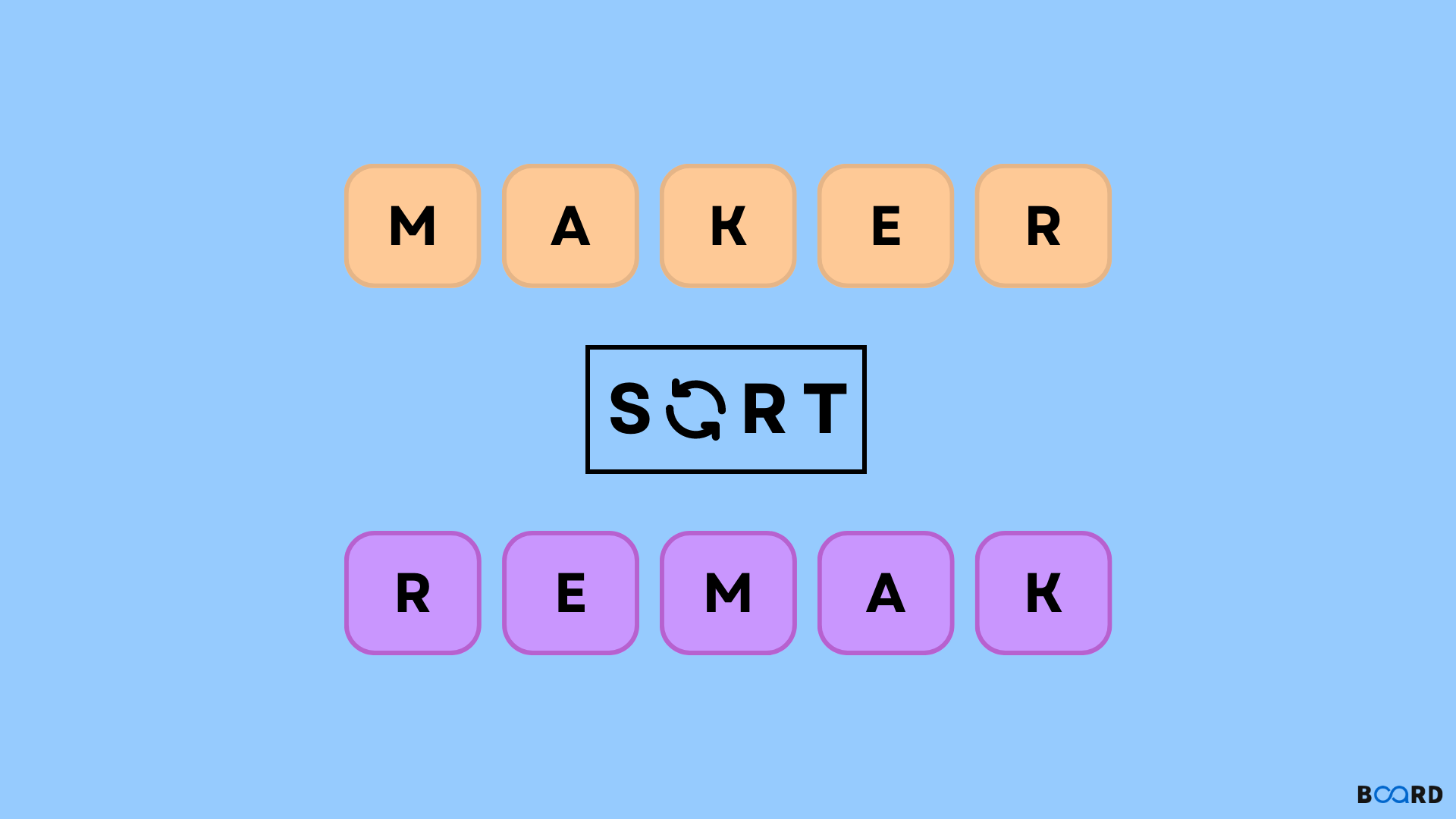String Sorting

# String Sort in Python## Methods to Sort a String

### Utilizing the Python Sorted() and Join() Methods

Python's sorted() function returns a "sorted list" of the iterable (list, tuple, set, etc.) object that was passed in as input. In our function, we can also define ascending or descending order.

The join() method unites all of the elements of an iterable (list, tuple, set, etc.) into a single string. Based on the supplied separator, it connects the string. Separator refers to the character that will be used to join the string; for example, a space, a dash, etc. Our join method merges the string with it based on the separator that has been supplied.

So, to sort our string, we can combine the sorted() and join() methods. A sorted list is returned by the sorted() function, while a joined list is created by the join() function.

Example:

 #python program to demonstrate sorting of a string using sorted and join() methoddef sort_string(s):    #First sort the string using sorted()    #store the list returned in ls    ls = sorted(s)    #join the list ls using join()    ans = ''.join(ls)    return ans#pass string to our functionprint(sort_string("python"))

Output:

 hnopty
write your code here: Coding Playground

### Using lambda, Reduce(), and Sorted()

Applying a certain function supplied as an argument to all list elements mentioned in the "sequence" argument is what the reduce(fun,seq) function does. In the "functools" module, this function is defined.

The lambda keyword is used to define anonymous functions (functions without names) in Python. Any number of parameters, but only one expression, can be passed to a lambda function. The expression is assessed and given a result. Let's examine how we can sort our string in Python using the sorted(), reduce(), and lambda functions.

Example:

 #Python program to sort a string using sorted() + reduce() + lambdaimport functoolsdef sort_string(str):    #First sort the string    s = sorted(str)    #Then concatenate the string using + operator    #followed by the reduce function to apply     #the concatenation on all the values    result = functools.reduce(lambda x, y: x + y, s )    return resultprint(sort_string("kolkata"))

Output:

 aakklot
write your code here: Coding Playground

### Using Python's While loop

Python's standard while loop function can also be used to sort strings. It is not, however, in any way, a recommended approach. You can simply comprehend this for the sake of education.

Example:

 #python program to demonstrate sorting of a string using while loopdef sort_string(mylist):    #list to store our result    ls = []    while mylist:        #append minimum element of the list of strings        ls.append(min(mylist))        #remove that minimum element from original string        mylist.remove(min(mylist))    return lsprint(sort_string(["python","java","c","ruby"]))

Output:

 ['c', 'java', 'python', 'ruby']
write your code here: Coding Playground

## Conclusion

• In Python, organizing a string into a specific order is referred to as sorting.
• Using sorted() to sort a string and join() to join the list that sorted returned, we can sort a string ().
• By iterating through the string and comparing them as they are sorted, we can also use bubble sort.
• By first using sort, then lambda to join the string, and then reduction to apply lambda to all the strings, sorted, lambda, and reduce can be used to sort a string in Python.
• By adding the smallest string to a list one at a time and removing it from the original string, the while loop can also sort a string. However, it is not a suggested approach.